# RS Aggarwal Solutions for Class 6 Chapter 10 Ratio, Proportion and Unitary Method Exercise 10A

The ratio of two non zero numbers a and b is the fraction a/b and we write it as ‘a:b’ read as ‘a is to b’. The first exercise of chapter 10 has problems which are solved based on ratio of numbers and the steps followed according to CBSE guidelines. RS Aggarwal Solutions are a major study material for the students in order to gain a better hold on the concepts. The students can use RS Aggarwal Solutions for Class 6 Chapter 10 Ratio, Proportion and Unitary Method Exercise 10A to improve their speed of solving which are important from exam point of view.

## Download PDF of RS Aggarwal Solutions for Class 6 Chapter 10 Ratio, Proportion and Unitary Method Exercise 10A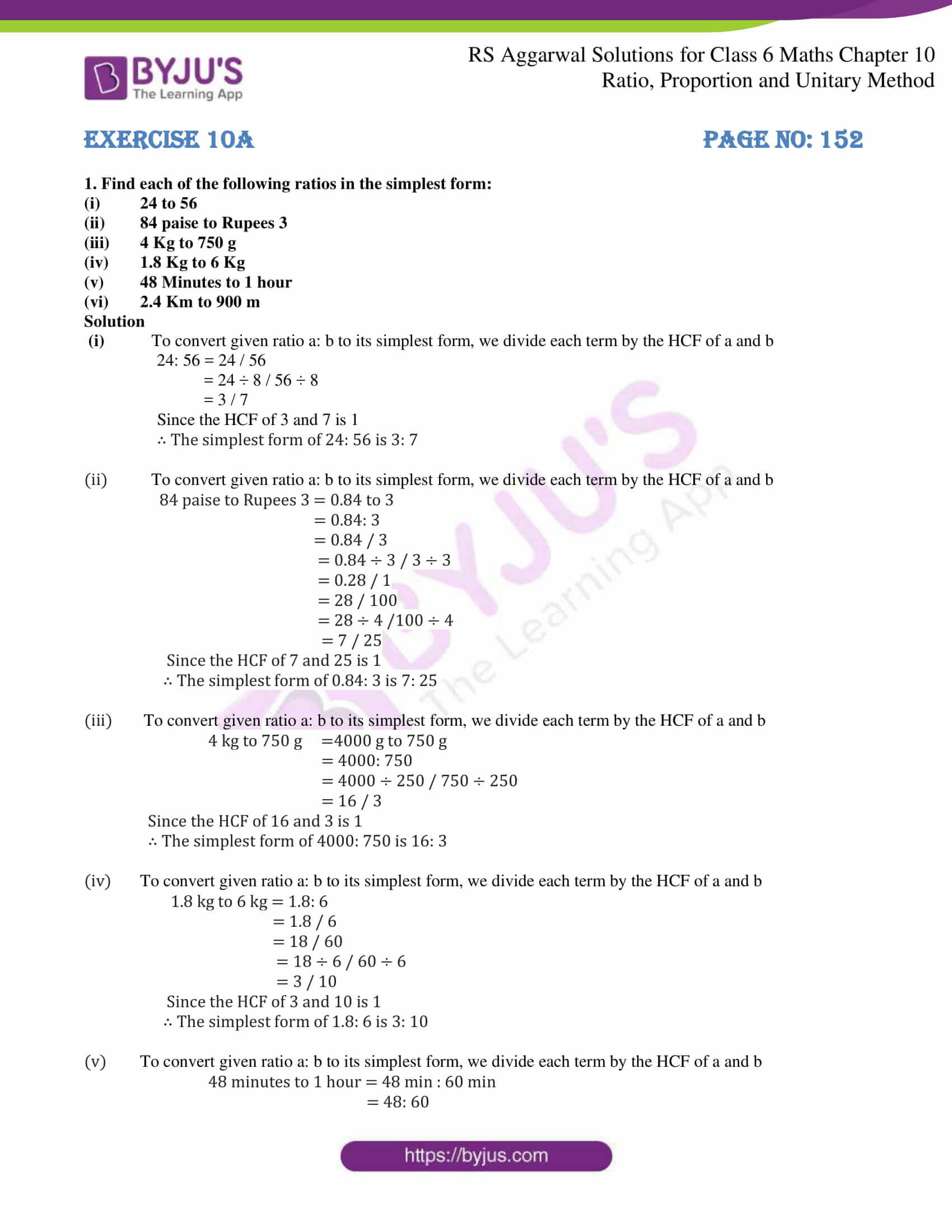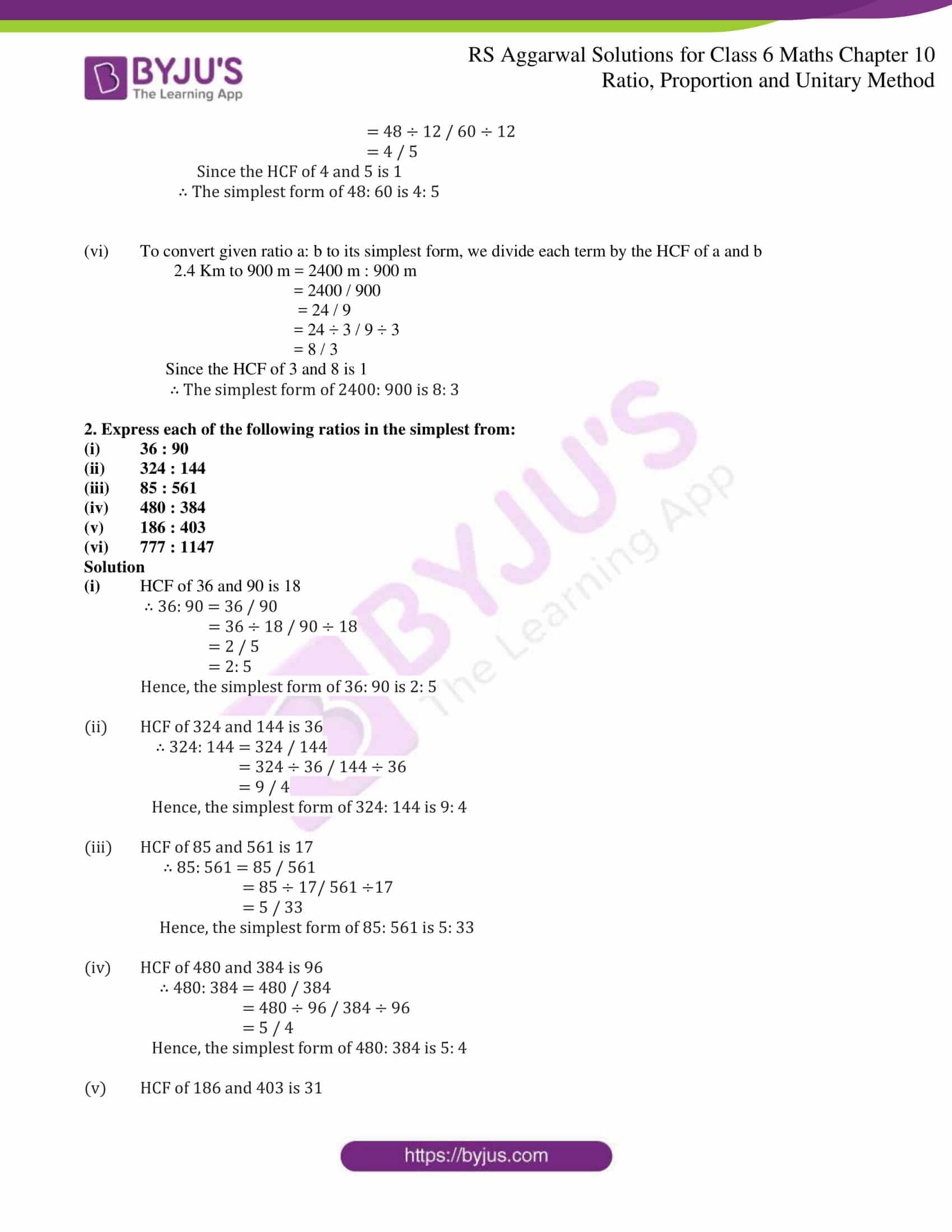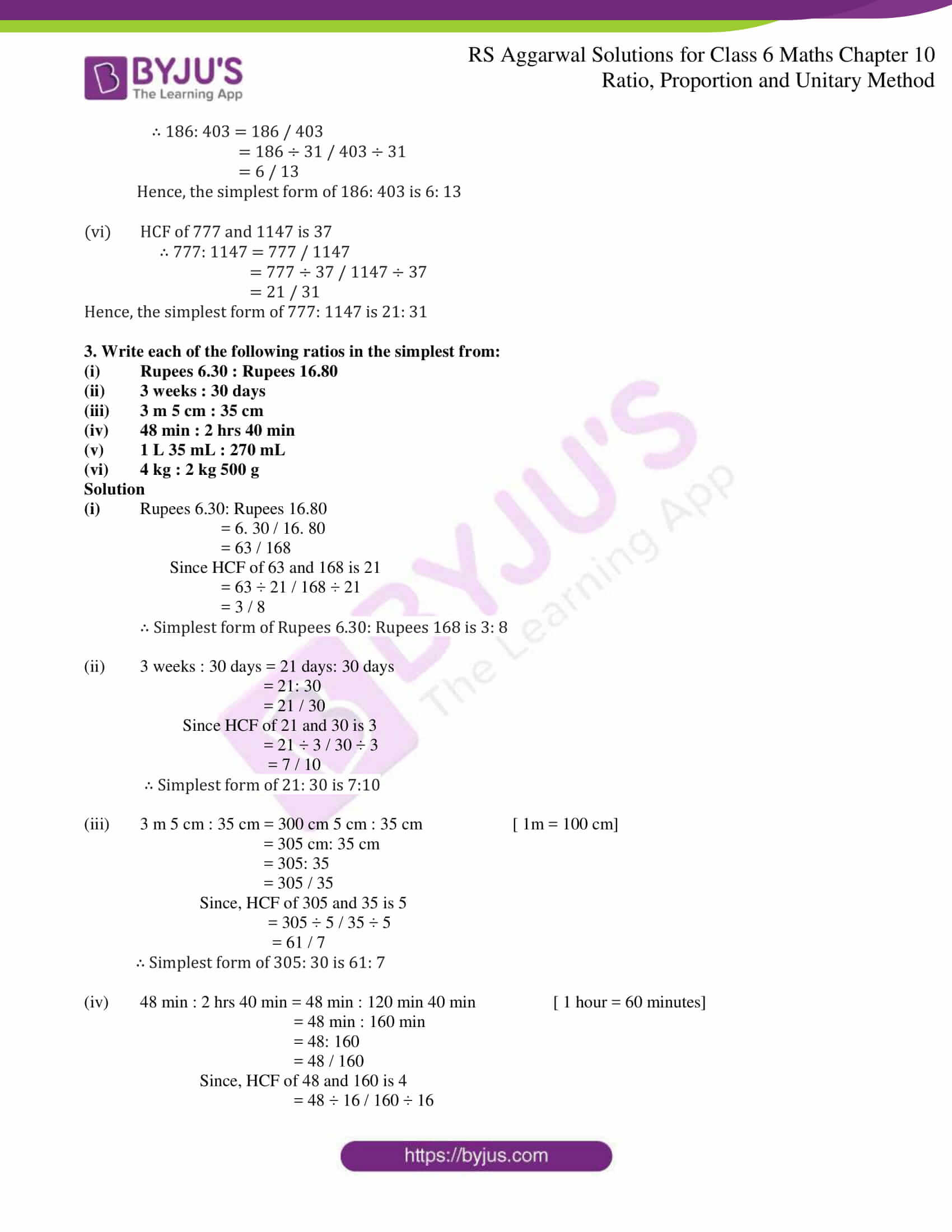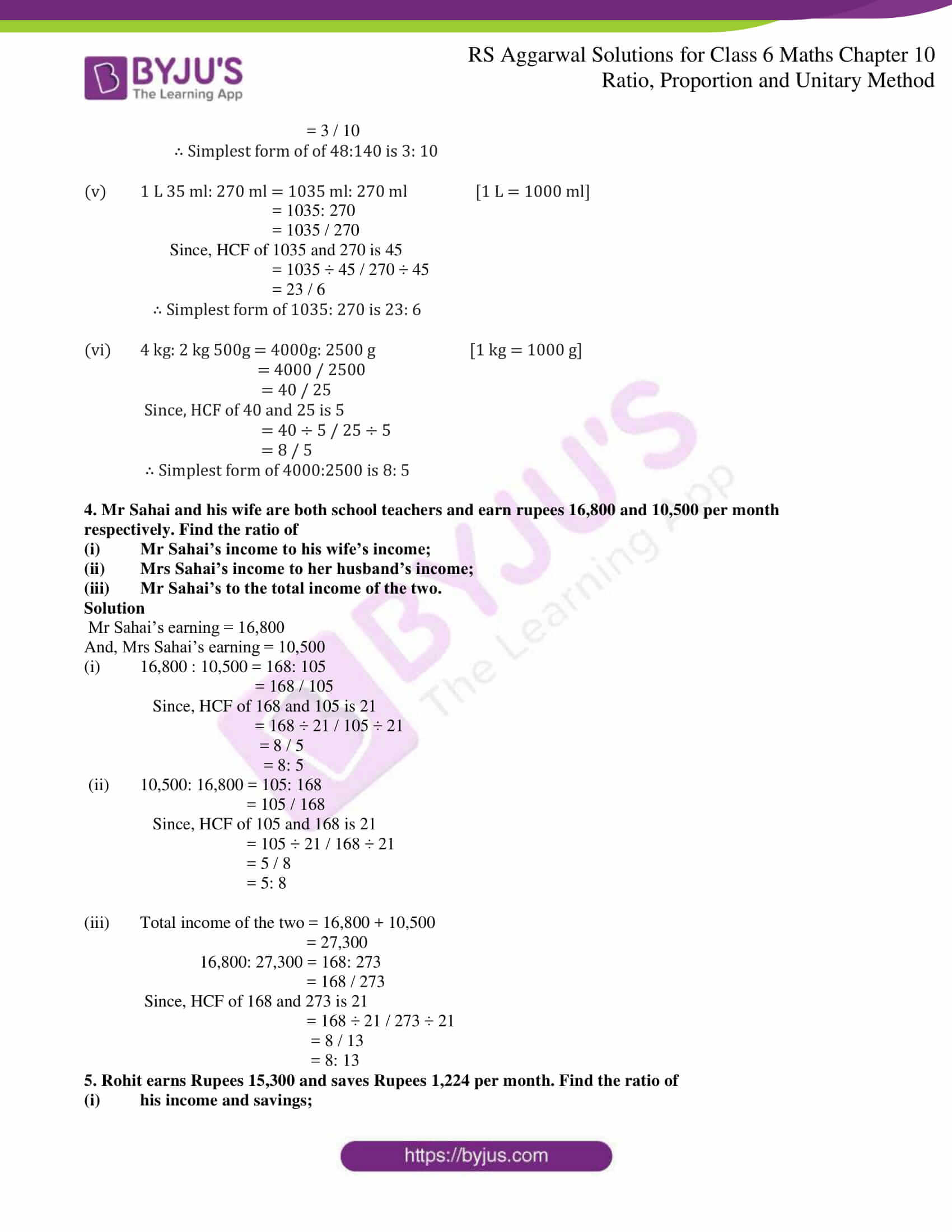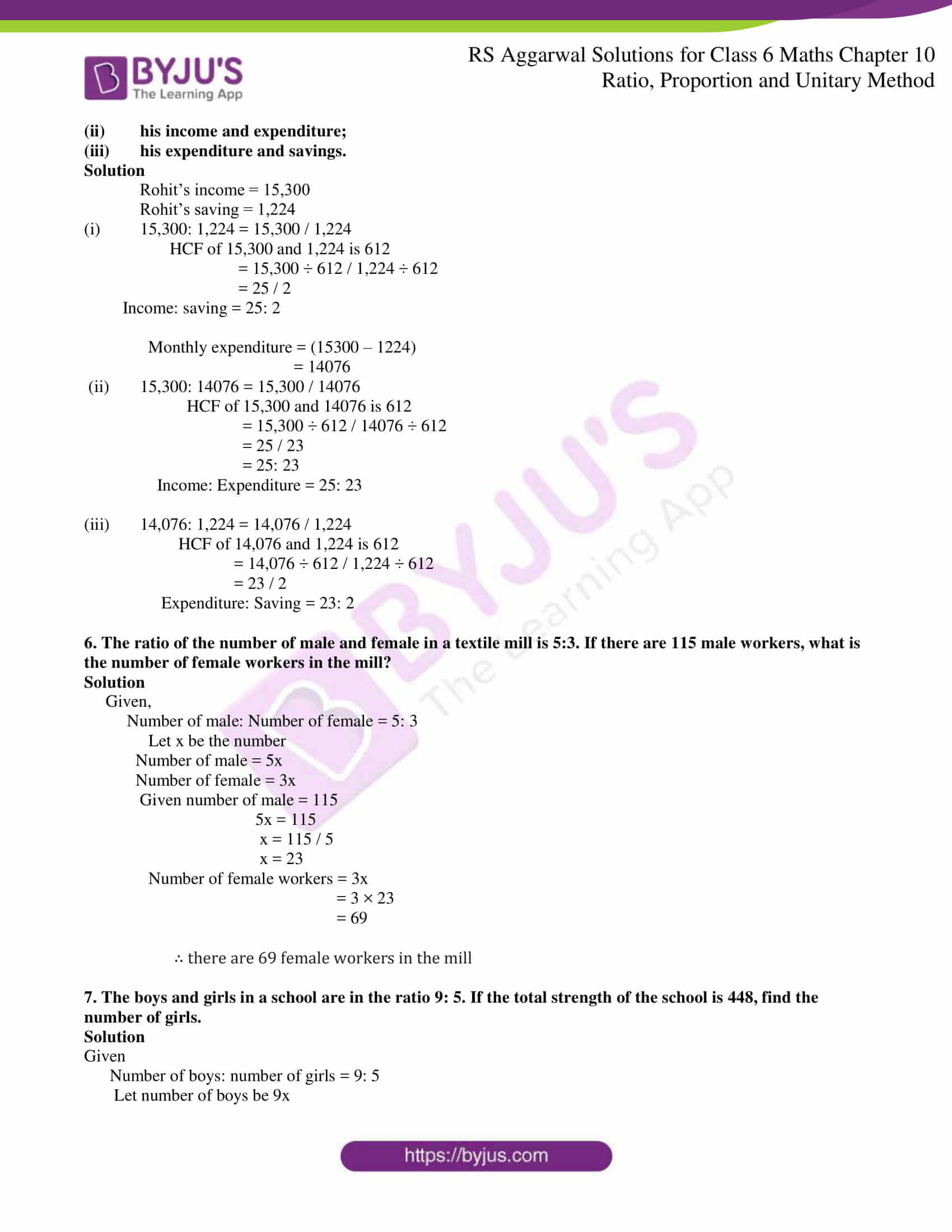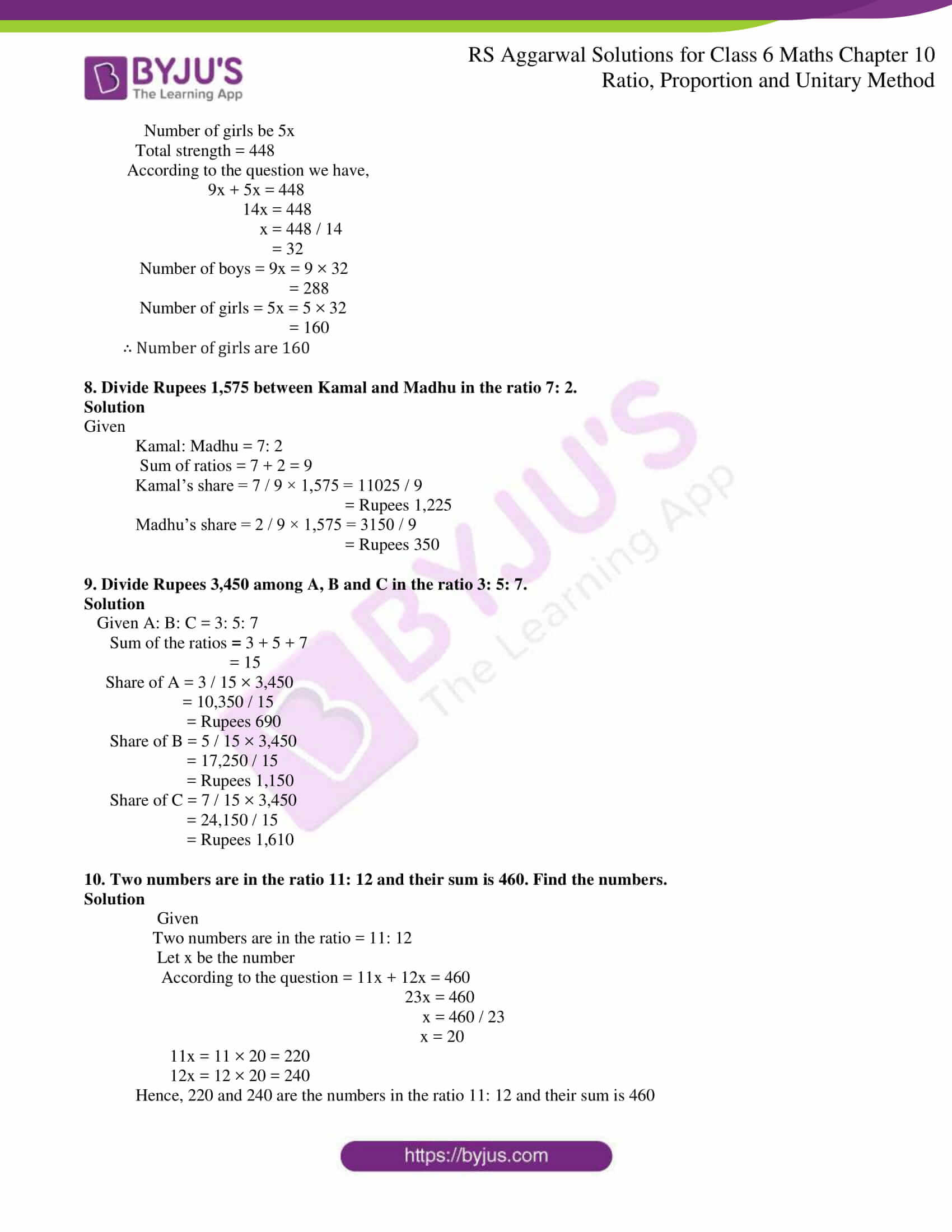### Access answers to Maths RS Aggarwal Solutions for Class 6 Chapter 10 Ratio, Proportion and Unitary Method Exercise 10A

1. Find each of the following ratios in the simplest form:

(i) 24 to 56

(ii) 84 paise to Rupees 3

(iii) 4 Kg to 750 g

(iv) 1.8 Kg to 6 Kg

(v) 48 Minutes to 1 hour

(vi) 2.4 Km to 900 m

Solution

(i) To convert given ratio a: b to its simplest form, we divide each term by the HCF of a and b

24: 56 = 24 / 56

= 24 ÷ 8 / 56 ÷ 8

= 3 / 7

Since the HCF of 3 and 7 is 1

∴ The simplest form of 24: 56 is 3: 7

(ii) To convert given ratio a: b to its simplest form, we divide each term by the HCF of a and b

84 paise to Rupees 3 = 0.84 to 3

= 0.84: 3

= 0.84 / 3

= 0.84 ÷ 3 / 3 ÷ 3

= 0.28 / 1

= 28 / 100

= 28 ÷ 4 /100 ÷ 4

= 7 / 25

Since the HCF of 7 and 25 is 1

∴ The simplest form of 0.84: 3 is 7: 25

(iii) To convert given ratio a: b to its simplest form, we divide each term by the HCF of a and b

4 kg to 750 g =4000 g to 750 g

= 4000: 750

= 4000 ÷ 250 / 750 ÷ 250

= 16 / 3

Since the HCF of 16 and 3 is 1

∴ The simplest form of 4000: 750 is 16: 3

(iv) To convert given ratio a: b to its simplest form, we divide each term by the HCF of a and b

1.8 kg to 6 kg = 1.8: 6

= 1.8 / 6

= 18 / 60

= 18 ÷ 6 / 60 ÷ 6

= 3 / 10

Since the HCF of 3 and 10 is 1

∴ The simplest form of 1.8: 6 is 3: 10

(v) To convert given ratio a: b to its simplest form, we divide each term by the HCF of a and b

48 minutes to 1 hour = 48 min : 60 min

= 48: 60

= 48 ÷ 12 / 60 ÷ 12

= 4 / 5

Since the HCF of 4 and 5 is 1

∴ The simplest form of 48: 60 is 4: 5

(vi) To convert given ratio a: b to its simplest form, we divide each term by the HCF of a and b

2.4 Km to 900 m = 2400 m : 900 m

= 2400 / 900

= 24 / 9

= 24 ÷ 3 / 9 ÷ 3

= 8 / 3

Since the HCF of 3 and 8 is 1

∴ The simplest form of 2400: 900 is 8: 3

2. Express each of the following ratios in the simplest from:

(i) 36 : 90

(ii) 324 : 144

(iii) 85 : 561

(iv) 480 : 384

(v) 186 : 403

(vi) 777 : 1147

Solution

(i) HCF of 36 and 90 is 18

∴ 36: 90 = 36 / 90

= 36 ÷ 18 / 90 ÷ 18

= 2 / 5

= 2: 5

Hence, the simplest form of 36: 90 is 2: 5

(ii) HCF of 324 and 144 is 36

∴ 324: 144 = 324 / 144

= 324 ÷ 36 / 144 ÷ 36

= 9 / 4

Hence, the simplest form of 324: 144 is 9: 4

(iii) HCF of 85 and 561 is 17

∴ 85: 561 = 85 / 561

= 85 ÷ 17/ 561 ÷17

= 5 / 33

Hence, the simplest form of 85: 561 is 5: 33

(iv) HCF of 480 and 384 is 96

∴ 480: 384 = 480 / 384

= 480 ÷ 96 / 384 ÷ 96

= 5 / 4

Hence, the simplest form of 480: 384 is 5: 4

(v) HCF of 186 and 403 is 31

∴ 186: 403 = 186 / 403

= 186 ÷ 31 / 403 ÷ 31

= 6 / 13

Hence, the simplest form of 186: 403 is 6: 13

(vi) HCF of 777 and 1147 is 37

∴ 777: 1147 = 777 / 1147

= 777 ÷ 37 / 1147 ÷ 37

= 21 / 31

Hence, the simplest form of 777: 1147 is 21: 31

3. Write each of the following ratios in the simplest from:

(i) Rupees 6.30 : Rupees 16.80

(ii) 3 weeks : 30 days

(iii) 3 m 5 cm : 35 cm

(iv) 48 min : 2 hrs 40 min

(v) 1 L 35 mL : 270 mL

(vi) 4 kg : 2 kg 500 g

Solution

(i) Rupees 6.30: Rupees 16.80

= 6. 30 / 16. 80

= 63 / 168

Since HCF of 63 and 168 is 21

= 63 ÷ 21 / 168 ÷ 21

= 3 / 8

∴ Simplest form of Rupees 6.30: Rupees 168 is 3: 8

(ii) 3 weeks : 30 days = 21 days: 30 days

= 21: 30

= 21 / 30

Since HCF of 21 and 30 is 3

= 21 ÷ 3 / 30 ÷ 3

= 7 / 10

∴ Simplest form of 21: 30 is 7:10

(iii) 3 m 5 cm : 35 cm = 300 cm 5 cm : 35 cm [ 1m = 100 cm]

= 305 cm: 35 cm

= 305: 35

= 305 / 35

Since, HCF of 305 and 35 is 5

= 305 ÷ 5 / 35 ÷ 5

= 61 / 7

∴ Simplest form of 305: 30 is 61: 7

(iv) 48 min : 2 hrs 40 min = 48 min : 120 min 40 min [ 1 hour = 60 minutes]

= 48 min : 160 min

= 48: 160

= 48 / 160

Since, HCF of 48 and 160 is 4

= 48 ÷ 16 / 160 ÷ 16

= 3 / 10

∴ Simplest form of of 48:140 is 3: 10

(v) 1 L 35 ml: 270 ml = 1035 ml: 270 ml [1 L = 1000 ml]

= 1035: 270

= 1035 / 270

Since, HCF of 1035 and 270 is 45

= 1035 ÷ 45 / 270 ÷ 45

= 23 / 6

∴ Simplest form of 1035: 270 is 23: 6

(vi) 4 kg: 2 kg 500g = 4000g: 2500 g [1 kg = 1000 g]

= 4000 / 2500

= 40 / 25

Since, HCF of 40 and 25 is 5

= 40 ÷ 5 / 25 ÷ 5

= 8 / 5

∴ Simplest form of 4000:2500 is 8: 5

4. Mr Sahai and his wife are both school teachers and earn rupees 16,800 and 10,500 per month respectively. Find the ratio of

(i) Mr Sahai’s income to his wife’s income;

(ii) Mrs Sahai’s income to her husband’s income;

(iii) Mr Sahai’s to the total income of the two.

Solution

Mr Sahai’s earning = 16,800

And, Mrs Sahai’s earning = 10,500

(i) 16,800 : 10,500 = 168: 105

= 168 / 105

Since, HCF of 168 and 105 is 21

= 168 ÷ 21 / 105 ÷ 21

= 8 / 5

= 8: 5

(ii) 10,500: 16,800 = 105: 168

= 105 / 168

Since, HCF of 105 and 168 is 21

= 105 ÷ 21 / 168 ÷ 21

= 5 / 8

= 5: 8

(iii) Total income of the two = 16,800 + 10,500

= 27,300

16,800: 27,300 = 168: 273

= 168 / 273

Since, HCF of 168 and 273 is 21

= 168 ÷ 21 / 273 ÷ 21

= 8 / 13

= 8: 13

5. Rohit earns Rupees 15,300 and saves Rupees 1,224 per month. Find the ratio of

(i) his income and savings;

(ii) his income and expenditure;

(iii) his expenditure and savings.

Solution

Rohit’s income = 15,300

Rohit’s saving = 1,224

(i) 15,300: 1,224 = 15,300 / 1,224

HCF of 15,300 and 1,224 is 612

= 15,300 ÷ 612 / 1,224 ÷ 612

= 25 / 2

Income: saving = 25: 2

Monthly expenditure = (15300 – 1224)

= 14076

(ii) 15,300: 14076 = 15,300 / 14076

HCF of 15,300 and 14076 is 612

= 15,300 ÷ 612 / 14076 ÷ 612

= 25 / 23

= 25: 23

Income: Expenditure = 25: 23

(iii) 14,076: 1,224 = 14,076 / 1,224

HCF of 14,076 and 1,224 is 612

= 14,076 ÷ 612 / 1,224 ÷ 612

= 23 / 2

Expenditure: Saving = 23: 2

6. The ratio of the number of male and female in a textile mill is 5:3. If there are 115 male workers, what is the number of female workers in the mill?

Solution

Given,

Number of male: Number of female = 5: 3

Let x be the number

Number of male = 5x

Number of female = 3x

Given number of male = 115

5x = 115

x = 115 / 5

x = 23

Number of female workers = 3x

= 3 × 23

= 69

∴ there are 69 female workers in the mill

7. The boys and girls in a school are in the ratio 9: 5. If the total strength of the school is 448, find the number of girls.

Solution

Given

Number of boys: number of girls = 9: 5

Let number of boys be 9x

Number of girls be 5x

Total strength = 448

According to the question we have,

9x + 5x = 448

14x = 448

x = 448 / 14

= 32

Number of boys = 9x = 9 × 32

= 288

Number of girls = 5x = 5 × 32

= 160

∴ Number of girls are 160

8. Divide Rupees 1,575 between Kamal and Madhu in the ratio 7: 2.

Solution

Given

Sum of ratios = 7 + 2 = 9

Kamal’s share = 7 / 9 × 1,575 = 11025 / 9

= Rupees 1,225

Madhu’s share = 2 / 9 × 1,575 = 3150 / 9

= Rupees 350

9. Divide Rupees 3,450 among A, B and C in the ratio 3: 5: 7.

Solution

Given A: B: C = 3: 5: 7

Sum of the ratios = 3 + 5 + 7

= 15

Share of A = 3 / 15 × 3,450

= 10,350 / 15

= Rupees 690

Share of B = 5 / 15 × 3,450

= 17,250 / 15

= Rupees 1,150

Share of C = 7 / 15 × 3,450

= 24,150 / 15

= Rupees 1,610

10. Two numbers are in the ratio 11: 12 and their sum is 460. Find the numbers.

Solution

Given

Two numbers are in the ratio = 11: 12

Let x be the number

According to the question = 11x + 12x = 460

23x = 460

x = 460 / 23

x = 20

11x = 11 × 20 = 220

12x = 12 × 20 = 240

Hence, 220 and 240 are the numbers in the ratio 11: 12 and their sum is 460